# Working with Microsoft Equation

Word
Microsoft Equation helps you add fractions, exponents, integrals, and so on to Word documents. You start building an equation by opening Microsoft Equation:

To insert an equation in your document, on the Insert tab, in the Symbols group, click the arrow next to Equation: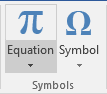You can use the vertical scroll bar in the Gallery to display additional equations (how to add an equation into the Gallery, see How to add your own equation to the Equation gallery). If you see what you want, click it to insert it at the current insertion point in the document. If you add it into an otherwise empty paragraph, the equation defaults to appearing in Display mode. If the equation is on the same line as text, it appears in Inline mode: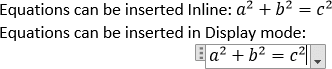• On the Insert tab, in the Symbols group, click the arrow next to Equation, and then click Insert New Equation,
• On the Insert tab, in the Symbols group, click the Equation button,
• Or simply press Alt+=.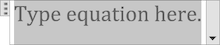Word 2016 opens the Design tab under Equation Tools: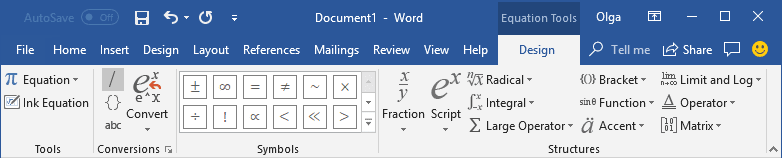Word 2016 provides two formats of equations: Professional and Linear: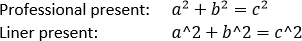By default, uses the Professional present, but if you ever need Linear, select the equation(s) you want to change, under Equation Tools, on the Design tab, in the Conversions group, in the Convert drop-down list, choose the appropriate option: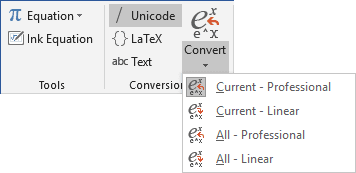Equation Tools Design tab contains dozens of equation templates. Within each button on the toolbar, there are several tools available. Simply click on a button to see the tools contained in each. How to create an equation step-by-step, see:

For more details about equations, see Setting font size and styles in an equation and Adjusting spacing and alignment in an equation.

See also this tip in French: Comment insérer des équations dans Microsoft Word.